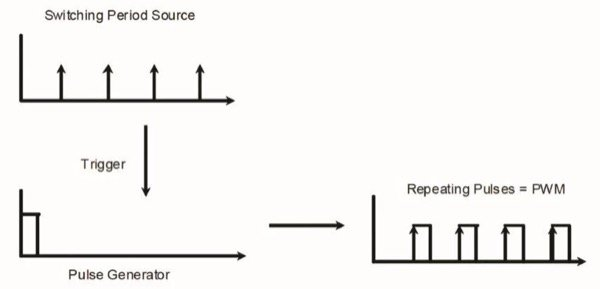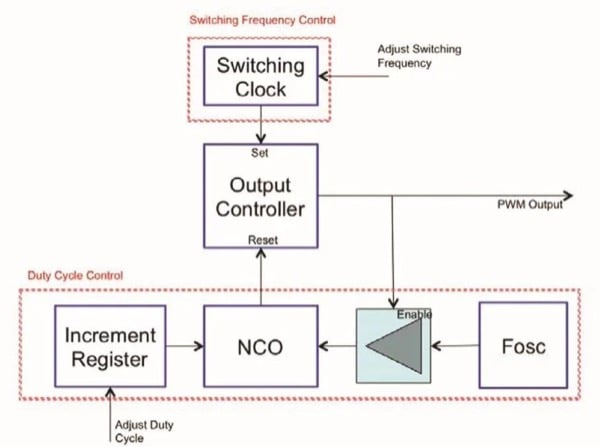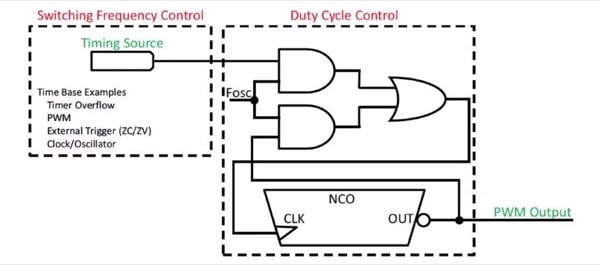# Combing the CLC and NCO to Implement a High-Resolution PWM

Many applications can function with pulse width modulation (PWM) resolutions of less than eight bits, but in some cases, such as when dimming lamps, a higher resolution is required due to the sensitivity of the human eye.

A conventional PWM uses a timer to produce a regular switching frequency and then uses a ripple counter to determine how many clocks the output is held high before the pulse ends. The output pulse width is adjusted to produce various duty cycle settings.

## Calculating Pulse Width Modulation (PWM) Resolution

The effective resolution (measured in bits) of a PWM can be calculated by taking the base-2 logarithm of the number of pulse width settings possible.

For a device running at 16MHz, the smallest duty cycle adjustment increment would be 62.5ns (one system clock). If the PWM is configured to run at a switching frequency of 200kHz (switching period of 5µs), 100 percent duty cycle will be achieved when the duty cycle register is set to 80 clocks (80 x 62.5ns = 5µs). This would make the effective PWM resolution only slightly more than six bits, as there are 80 steps to choose from. This is because one system clock divides into one period 80 times.

Knowing there are 80 possible duty cycle steps, a precise value for the resolution of the PWM can be calculated.

A PWM running from a 16MHz clock, which has a ten-bit duty cycle register, will start losing resolution due to this limitation at a switching frequency of 15.6kHz. For higher PWM switching frequencies, the duty cycle will reach 100 percent before all of the steps in the ten-bit duty cycle register have been used, and for all the remaining values the output will simply remain at 100 percent duty cycle. The frequency at which this point is reached can also be calculated.

In most PWM applications, the PWM is switched at a much higher frequency than the output can ever change. By filtering this PWM signal using a low-pass filter, the desired output is obtained. The filter removes the high-frequency switching components of the PWM by essentially calculating the average value of the PWM signal, and presents this as the output.

For example, in a switching power supply, the output voltage will be directly proportional to the duty cycle. The consequence of this is the smaller the adjustment to the PWM duty cycle, the smaller the resulting change to the output, leading to more precise control of the output.

From a control systems point of view, making small adjustments to the output effectively lowers the quantization gain introduced by the PWM. In control systems, this lowering of the gain helps ensure the stability of the system.

## PWM Design

As shown in Figure 1, in principle, a PWM is created by the combination of two parameters – a repeating trigger, which determines how often the switching period or switching frequency are pulsed, and a single-pulse generator, which determines how wide the pulse is (the duty cycle).Figure 1: PWM construction

To achieve an increase in the effective PWM resolution, the Numerically Controlled Oscillator (NCO) peripheral on a microcontroller, such as a Microchip PIC device, can be used to create a monostable circuit, which is one that gives a single pulse of fixed duration when triggered. The NCO will generate a signal that varies between two values in a defined proportion, creating an average pulse width, which is somewhere between two system clocks. The PWM signal pulse width will vary through jitter and dither by one clock period, with the proportion or ratio of the variation precisely determined by the NCO configuration.

In any application where the output is producing an average value, such as average power transfer to the load in SMPS or lighting applications, the variation in pulse width will be perfectly acceptable because the average pulse width is accurately controlled.

By itself, the NCO peripheral cannot produce a PWM signal, but its behavior can be changed by adding some logic using a Configurable Logic Cell (CLC) to produce a PWM output. This can be achieved by using the conventional PWM as a clock source to trigger the PWM period and use the NCO to determine the pulse width.

Any number of clock sources can be used, for example, timers or even external signals, and in some applications, an external trigger can be used to start the pulses, such as a zero-current detection circuit for power supplies. A simplified block diagram of this is shown in Fig. 2.Figure 2: the NCO-based principle of operation

The control logic in the CLC is used to set an output when the switching clock indicates it is time for the next pulse, and clear this output to complete the pulse once the NCO overflows.

## Implementation of NCO and CLC

An implementation of this design using the NCO and CLC is shown in Fig. 3. For this design, the NCO is placed in pulse frequency mode, in which a short pulse is produced when the NCO overflows.Figure 3: PWM implementation using CLC and NCO

When the system begins, the NCO output is low because it is waiting for enough clocks to count until it overflows and produces a pulse. This low output signal is inverted so the PWM output becomes high. This will supply a high-speed clock back into the NCO clock pin.

The PWM output will remain high until the accumulator overflows and the NCO output changes. This will cause it to stop producing the clock needed to run the NCO. At this point, the NCO is stuck high until it can get the clocks needed to finish its pulse. The PWM output is now low.

The timing source will then pulse high when the next period begins, feeding the high-speed clock back to the NCO.

The NCO uses these few clocks to finish the pulse, and then the output toggles back to the low position where it starts the process over from the beginning. The amount of time it takes the NCO to overflow will depend on the remainder left in the accumulator after the last overflow, as well as the increment register. Due to the accumulation of remainders, the pulse will sometimes be one system clock shorter than usual. By controlling how often this happens by setting the increment register, the exact average pulse width can be controlled.

The calculation of the pulse width will be according to the NCO overflow frequency calculation. The average overflow frequency of the NCO will determine the average output pulse width produced.

The NCO is designed to give linear control over frequency. The control over pulse width is subsequently not linear. The result is that the effective resolution of the PWM is not constant over the entire range from 0 to 100 percent duty cycle.

For every duty cycle setting, the effective resolution at this particular point can be calculated and then plotted on a graph. This curve will look different depending on what the switching frequency is, the pulse width is adjusted independently from the switching frequency.

## Conclusion

Conventional PWMs start losing effective resolution at relatively low switching frequencies. For applications where the switching frequencies have to be fairly high, and having as much PWM resolution as possible at these frequencies is necessary, the NCO can be used with the CLC to create a very high-resolution PWM output.

The smallest incremental change in pulse width achievable by a conventional PWM with a 16MHz system clock speed would be 62.5ns. If the fastest available PWM clock is a quarter the frequency of the oscillator (Fosc), then this increases to 250ns.

On the same device, a PWM with an incremental pulse width change of as little as 15ps can be constructed using this technique.

Even if the requirement is not primarily high resolution, this method may still be attractive for a number of applications, adding an additional PWM to the capability of the device, or having a constant on-off time variable frequency PWM, where the pulse is triggered externally as required when doing zero current switching in high-efficiency power converters.